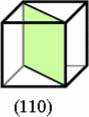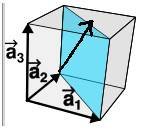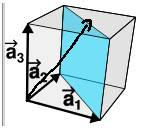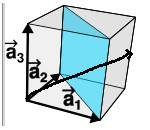# Solid State Physics - Miller Planes/Indices and Finding a

ChrisJ

## Homework Statement

Q3a [/B]
Copper (Cu) crystallises in a face-‐centre cubic (fcc) structure (with a basis of one copper
atom). Draw a diagram of the unit cell of Cu, on your diagram indicate the (1, 1, 0) lattice plane and the [2, 2, 1] lattice direction.

Q3b: Calculate the angle between the [2,2,1] and [0,0,1] lattice directions

Q3c:
A sample of magnesium oxide, MgO, is investigated using x-‐rays of wavelength 0.154 nm. The (2,2,0) Bragg peak is observed at a diffraction angle of 2θ = 59.4°. Calculate the cubic unit cell parameter a for MgO.

## Homework Equations

##2d \sin{\theta} = n \lambda ##
and maybe ## d = \frac{a}{\sqrt{h^2+l^2+k^2}} ## ?

## The Attempt at a Solution

This is not for coursework or homework, but for preparation for an exam and this was a question on a past exam paper (which we don't have solutions for). But posted here as thought its where it still needs to go, but was not sure if to post here or in the introductory physics thread, this is part of a 2nd (of 3) years BSc degree. Albeit this is one of the easy questions on the paper.

Q3a:
I am familiar with sketching in the miller planes and lattice directions for a simple cubic structure (aka primitive cubic), so I guess my main question is whether it is the same for a FCC? I have done a lot of searching online on this and have not found anything concrete, but have seen or two people saying it is the same, but my initial instinct was that its not.

If It is the same, then the (1,1,0) plane is just as belowAnd for the direction of [2,2,1] of, I am not the best at drawing and its easier to just explain it than draw it and take a photo and upload etc. But it would start at the origin, and go through the "roof" of the cube dead centre (the middle of the square roof), and if there were two cubes stacked upon each other would point towards the very top right corner at the "front" (the bit "closest" to the screen).

Q3b: Again if its the same process as for simple cubic, then surely it is just using the definition of the dot/scalar product, and would be 70.5°.

Q3c:
The main reason I posted this question was for this part as I had never come across the second equation I put in the relevant equations section; plus was not sure about just using 1 for n, or is n quite literally the |(2,2,0)| I have used (that makes more sense to me)?

But I just combined the two equations to get
$a=\frac{\lambda (\sqrt{h^2+l^2+k^2)}}{2 \sin{\theta}} \\ a=\frac{(1.54 \times 10^{-10}) (\sqrt{2^2+2^2)}}{2 \sin{(59.4/2)}} \\ a = 4.38 \times 10^{-10} = 4.38 Å$

Any help is much appreciated!

Thanks for the thread! This is an automated courtesy bump. Sorry you aren't generating responses at the moment. Do you have any further information, come to any new conclusions or is it possible to reword the post? The more details the better.

Homework Helper
Gold Member
Hello, Chris. Welcome to PF!
Since no one has responded to your post yet, I will try to help. (I'm not too familiar with solid state physics.)

Q3a. For the FCC lattice there is a distinction between a primitive unit cell and a conventional unit cell. See http://www.physics-in-a-nutshell.com/article/11#conventional-unit-cell. I suspect that in this problem you are meant to consider the conventional unit cell, which is a cube.
In your figure for the (1, 1, 0) plane, I think you should include the lattice vectors a1, etc. to show your choice of orientation.
I do not follow your description of the direction [2, 2, 1].

Q3c. I think your answer of 4.38 Å is correct. Using n = 1 with (2, 2, 0) is equivalent to using n = 2 with (1, 1, 0).

•ChrisJ
ChrisJ
Hello, Chris. Welcome to PF!
Since no one has responded to your post yet, I will try to help. (I'm not too familiar with solid state physics.)

Q3a. For the FCC lattice there is a distinction between a primitive unit cell and a conventional unit cell. See http://www.physics-in-a-nutshell.com/article/11#conventional-unit-cell. I suspect that in this problem you are meant to consider the conventional unit cell, which is a cube.
In your figure for the (1, 1, 0) plane, I think you should include the lattice vectors a1, etc. to show your choice of orientation.
I do not follow your description of the direction [2, 2, 1].

Q3c. I think your answer of 4.38 Å is correct. Using n = 1 with (2, 2, 0) is equivalent to using n = 2 with (1, 1, 0).

Thanks for taking the time to reply :) Thanks for the link, I hadnt come across that one yet and it seems to explain/answer what I was looking for.

Yeah sorry my explanation for the lattice direction of [2,2,1] was rather convoluted, plus I think had it wrong anyway. I am not too sure of the convention, I may be wrong but I'm sure my lecturer switched using different conventions, or direction of lattice vectors/ place of "origin", and in the notes the diagrams/figures don't always have them labelled which confuses things; that image from my OP was taken from his lecture notes.

The direction of [2,2,1], when choosing the lattice vectors as shown below, is either this below: (the plane is the (1,1,0) plane and the lattice direction was drew on over the top, it is obvs supposed to be straight, in this one the line is on the plane, hitting the "roof" in the centre.Or this way:Thanks :)

Homework Helper
Gold Member
Your drawing for the vector [2, 2, 1] doesn't look correct to me. Shouldn't this be in the same direction as a vector with x-component of 2, y-component of 2, and z-component of 1? Here, "x-component" means parallel to a1, etc.

ChrisJ
Your drawing for the vector [2, 2, 1] doesn't look correct to me. Shouldn't this be in the same direction as a vector with x-component of 2, y-component of 2, and z-component of 1? Here, "x-component" means parallel to a1, etc.

Oh bugger, yeah, I have drawn [1,1,2] (I think) not [2,2,1] haha. I think I got it now, il post the img in a sec.

Last edited:
ChrisJ
Your drawing for the vector [2, 2, 1] doesn't look correct to me. Shouldn't this be in the same direction as a vector with x-component of 2, y-component of 2, and z-component of 1? Here, "x-component" means parallel to a1, etc.

Ok here we go...Essentially [1,1,1/2], but since the convection is to multiply by smallest no to get all integers it is the same as [2,2,1], at least that's how I think it is? But I was not sure if the standard convention for lattice directions when using the lattice vectors like in the pic above, is to start all lattice direction from where the lattice vectors originate from?

Either that is the case, or when dealing with Miller planes, my lecturer has the lattice vectors like above, but when doing lattice directions he has them for example originating from where a2 ends, but in the diagrams does not indicate the vectors at all haha. The notes are so confusing.

Homework Helper
Gold Member
OK, your drawing looks good for both the vector and the plane.

I don't think it matters what point the vector is drawn from as long as it has the correct direction. I also don't think it matters how long you draw the vector if you just want to indicate the direction.

•ChrisJ
ChrisJ
OK, your drawing looks good for both the vector and the plane.

I don't think it matters what point the vector is drawn from as long as it has the correct direction. I also don't think it matters how long you draw the vector if you just want to indicate the direction.

Ok thanks for your help :)